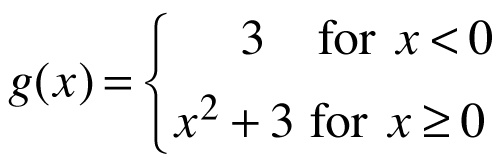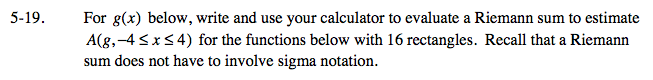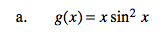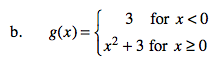### Home > CALC > Chapter 5 > Lesson 5.1.2 > Problem5-19

5-19.
1. For g(x) below, write and use your calculator to evaluate a Riemann sum to estimate A(g, −4 ≤ x ≤ 4) for the functions below with 16 rectangles. Recall that a Riemann sum does not have to involve sigma notation. Homework Help ✎

1. g(x) = x sin2 x

2.General form of a right-endpoint Riemann sum:Your Riemann sum will need to split into two parts.

A(g) = 42.25